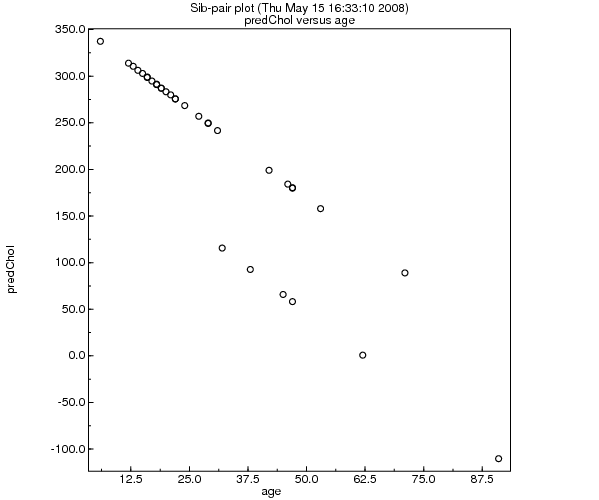# Sib-pair Command: predict

 Class Analysis and data manipulation command Name predict Arguments on ...[to]... [complete_obs].

Replace quantitative trait with the predicted value from the multivariate regression on the list of predictors (which may include the average allele length of a marker locus). The com option means only individuals with no missing values for any of the listed traits will be updated. Otherwise, missing values are replaced with the sample mean for that phenotype, when calculating the predicted value.

Example:

```>> predict predChol =  age ldl

------------------------------------------------
Linear regression analysis of trait "predChol"
------------------------------------------------

Variable         Beta    Stand Error        t-Value
-----------------------------------------------------
Intercept      238.1684        43.0137         5.5370 ***
age             -3.8292         1.5887         2.4102 *
ldl*2          120.6935        35.4199         3.4075 **

No. usable observations =     27      (  45.0%)
Model Mean Square       =  74284.9308 (df=   2)
Mean Square Error       =  11872.6848 (df=  24)
Multiple R**2           =      0.3427
Akaike Inf. Criterion   =      9.5301

Wrote     60 predicted values to predChol.

>> plot age predChol

```<< (residuals) Up to index >> (impute)